# Trigonometry: Basic and More

This is a collection of worksheets for the sine, cosine and tangent function that start at the very basic definitions and work through to the definitions involving the unit circle and radians. They work on an ipad and a tablet. I have put them on these wikispaces pages:
•### Simple Sine

•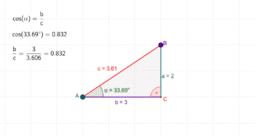### Simple Cosine

•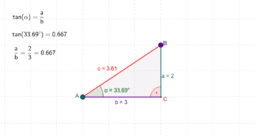### Simple Tangent

•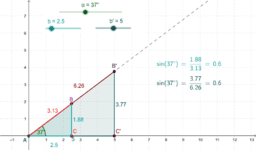### Sine Values and Nested Triangles

•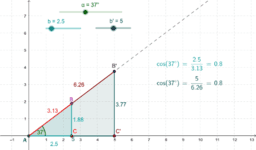### Cosine Values and Nested Triangles

•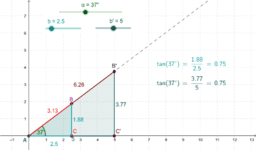### Tangent Values and Nested Triangles

•### Sin(x) & Unit Circle - Degrees

•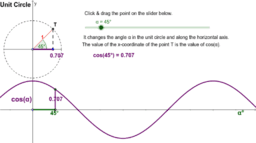### Cos(x) & Unit Circle - Degrees

•### Tan(x) & Unit Circle - Degrees

•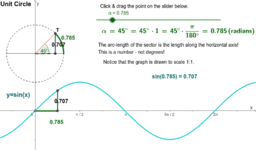### Sin(x) & Unit Circle - Radians

•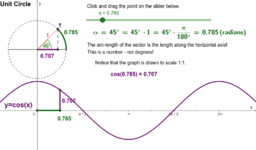### Cos(x) & Unit Circle - Radians

•